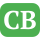CppBuzz.com

# C++ Program Development Life Cycle

When we want to develop a program using any programming language, we follow a sequence of steps. These steps are called phases in program development. The program development life cycle is a set of steps or phases that are used to develop a program in any programming language. Generally, program development life cycle contains 6 phases, they are as follows….

• Problem Definition
• Problem Analysis
• Algorithm Development
• Coding & Documentation
• Testing & Debugging
• Maintenance

## 1. Problem Definition

In this phase, we define the problem statement and we decide the boundaries of the problem. In this phase we need to understand the problem statement, what is our requirment, what should be the output of the problem solution. These are defined in this first phase of the program development life cycle.

## 2. Problem Analysis

In phase 2, we determine the requirements like variables, functions, etc. to solve the problem. That means we gather the required resources to solve the problem defined in the problem definition phase. We also determine the bounds of the solution.

## 3. Algorithm Development

During this phase, we develop a step by step procedure to solve the problem using the specification given in the previous phase. This phase is very important for program development. That means we write the solution in step by step statements.

## 4. Coding & Documentation

This phase uses a programming language to write or implement actual programming instructions for the steps defined in the previous phase. In this phase, we construct actual program. That means we write the program to solve the given problem using programming languages like C, C++, Java etc.,

## 5. Testing & Debugging

During this phase, we check whether the code written in previous step is solving the specified problem or not. That means we test the program whether it is solving the problem for various input data values or not. We also test that whether it is providing the desired output or not.

## 6. Maintenance

During this phase, the program is actively used by the users. If any enhancements found in this phase, all the phases are to be repeated again to make the enhancements. That means in this phase, the solution (program) is used by the end user. If the user encounters any problem or wants any enhancement,then we need to repeat all the phases from the starting, so that the encountered problem is solved or enhancement is added.